An integer which is a measure of the singularity of the algebraic variety at that point. The multiplicity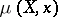of a varietyat a pointis defined to be the multiplicity of the maximal ideal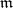in the local ring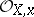. The multiplicity ofatcoincides with the multiplicity of the tangent coneat the vertex, and also with the degree of the special fibre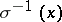of a blow-upofat, where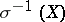is considered to be immersed in the projective space(see ). One has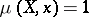if and only ifis a non-singular (regular) point of. Ifis a hypersurface in a neighbourhood of(i.e.is given by a single equation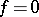in an affine space), thenis identical with the numbersuch that, whereis the maximal ideal in the local ring. The multiplicity does not change whenis cut by a generic hypersurface through. If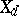denotes the set of pointssuch that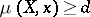, thenis a closed subset (a subvariety).